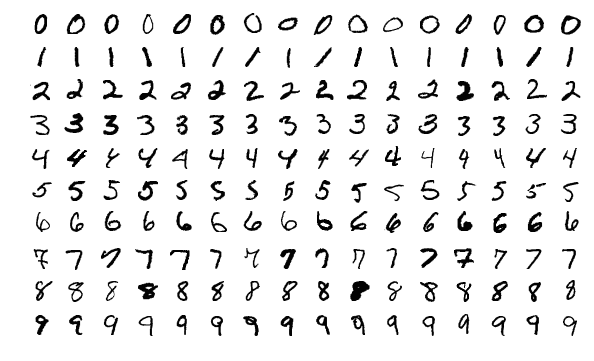# Exploring the Classic MNIST: A Benchmark for Machine Learning Models

In the realm of machine learning and pattern recognition, the MNIST dataset stands as a benchmark for algorithms and methods. It’s akin to the `hello world` of machine learning for classification tasks. Before delving into the technicalities of multi-class classification, let’s embark on a journey to fully understand the MNIST dataset, its uses, and its significance.

# What is the MNIST Dataset?

MNIST, which stands for the Modified National Institute of Standards and Technology database, is a large collection of handwritten digits commonly used for training various image processing systems. The dataset contains 70,000 images of handwritten digits from 0 to 9, each a 28x28 pixel grayscale image. For simplicity and efficiency in training, these images are often flattened into a 1D array of 784 pixels.## Usage and Benchmarks

The primary use of the MNIST dataset is to provide a straightforward dataset for algorithms to learn number recognition—making it a gateway into the field of machine learning for beginners. As for benchmarks, many algorithms have been tested on MNIST, achieving varying levels of accuracy. Simple models like k-nearest neighbors can reach about 97% accuracy, while more sophisticated neural networks, including MLPs, have achieved over 99% accuracy on the dataset.

# Multi-Class Classification and MLPs

MNIST is a classic example of a multi-class classification problem, where the task is to classify the images into one of the ten possible classes (digits 0 through 9). An MLP, or Multi-Layer Perceptron, is a type of neural network that’s well-suited for this kind of task. It consists of an input layer, one or more hidden layers, and an output layer.

## Label Encoding with One-Hot

In multi-class classification, it’s essential to encode categorical labels in a way that the machine learning model can understand — this is where one-hot encoding comes into play. For the MNIST dataset, this means converting the numeric label of each image into a vector of length 10, where the index corresponding to the digit is marked with a 1, and all other indices are 0. For example, the digit 3 would be encoded as [0, 0, 0, 1, 0, 0, 0, 0, 0, 0].

## Normalization of Input Data

The input data normalization is a crucial step in the preprocessing of data for neural networks. For MNIST, normalization involves scaling the pixel values from their original range of 0-255 to a range of 0-1 by dividing by 255. This process helps in speeding up the learning process and reaching better performance because neural networks work better with small input values.

## Understanding the Output Vector

The output of an MLP for the MNIST dataset is a vector of probabilities, each representing the network’s confidence that the image belongs to one of the ten digit classes. The predicted label is then simply the index of the highest probability in this vector.

## Softmax Function

The softmax function is a vital component when dealing with multi-class classification in neural networks. It converts the raw output scores (also known as logits) into probabilities by exponentiating and normalizing them, ensuring that the output probabilities sum up to one. Using softmax in the output layer of an MLP ensures a more interpretable and normalized prediction.

## Predicting Without Softmax

It’s possible to predict the label without applying softmax, especially if we are only interested in the most likely class. Since the softmax function preserves the order of the logits (i.e., if one logit is larger than another, it will still be larger after softmax), we can simply take the index of the highest logit to predict the class without converting logits to probabilities.

# Conclusion

The MNIST dataset serves as a fundamental benchmark in the machine learning community, allowing for the exploration of classification algorithms. By implementing MLPs for this dataset, one not only grasps the nuances of machine learning workflows, such as preprocessing and encoding but also gains the foundational knowledge required for tackling more complex multi-class classification problems. As we build and iterate upon these systems, we edge closer to creating models that aren’t just academic exercises but powerful tools for interpreting and understanding the visual world.

This blog post covers the MNIST dataset and the basics of multi-class classification, paving the way for readers to appreciate the nuances of neural network training and application. It provides an understanding of the key preprocessing steps necessary for successful model training and touches on the details of output interpretation, setting a foundation for more advanced topics in machine learning.Happy coding!

Share: